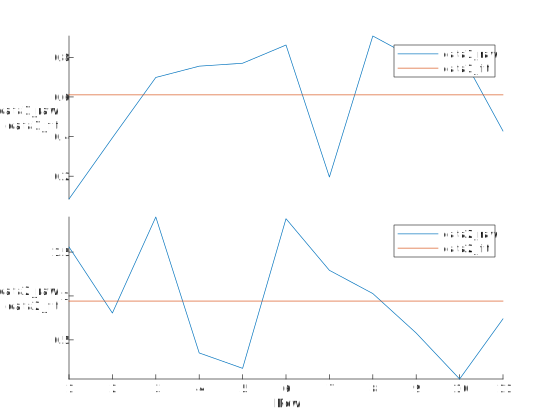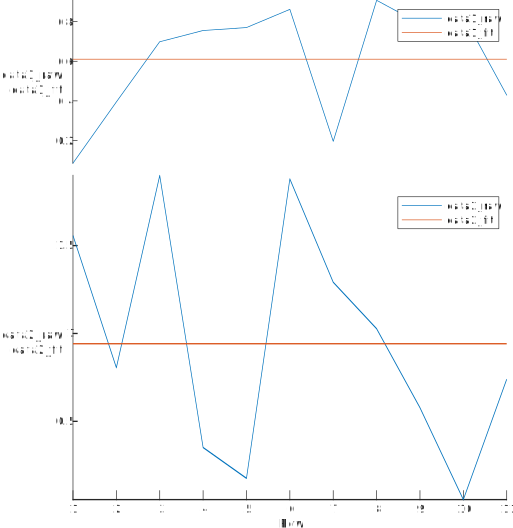# How do I change the size of subplots in stackedplot

14 views (last 30 days)
David Duncan on 9 Jun 2020
Edited: Adam Danz on 24 Nov 2020
I am trying to plot a series of related data together (sharing the same x-axis). Most sets of the data cover a very similar y range (0-1), but one of the data sets cover a comparably larger range (0-2). I desire each plot to be on its own y-axis, but with a shared x-axis, but I'd like the size of each subplot to match the total y-range that the respective data covers. So my script looks something like:
data1_raw = rand(11,1);
data1_fit = repmat(mean(data1_raw),11,1);
data2_raw = 2*rand(11,1);
data2_fit = repmat(mean(data2_raw),11,1);
t = table(data1_raw,data1_fit,data2_raw,data2_fit);
s = stackedplot(t,{{'data1_raw','data1_fit'},{'data2_raw','data2_fit'}});s.AxesProperties.plot_start = [0 0.33] %set the start position of each sub plot
What I get from this is the below plot:What I want is this plot:jessupj on 9 Jun 2020
basically, you'd do this via:
subplotX = @(m,n,p) subtightplot (m, n, p, [0.01 0.05], [0.1 0.01], [0.1 0.01]);
subplotX(3,1,1)
% plot first fig...
subplotX(3,1,[2 3])
% plot second fig...
David Duncan on 15 Jun 2020
That does indeed look like a good solution to my problem, thank you. Shame there doesn't appear to be any flexibility with the Mathwork's stackedplot though.

Ayush Gupta on 12 Jun 2020
MATLAB has hold on feature which allows to plot multiple graphs on the same figure. Refer to the following link to know more about hold on:
A Use case example:
plot(t.data1_raw);
hold on
plot(t.data1_fit);
plot(t.data2_raw);
plot(t.data2_fit);
hold off

jessupj on 12 Jun 2020
this isn't a question about plotting multiple data on a common axis; it is a question about sizing of subplots
David Duncan on 15 Jun 2020
I would argue it is about plotting multiple data on the same X-axis, but different Y-axis and being able to control the respective sizes of the y-axes, but it can indeed be achieved by resizing subplots and simply making sure that the limits of each x-axis are the same.
(But indeed it is not a "hold" issue).

Adam Danz on 19 Nov 2020
Edited: Adam Danz on 24 Nov 2020
You can get the axis handles of stackedplot using the undocumented NodeChildren property. Use flipud to set the axis handles in order of the axes as they appear in the plot.
s = stackedplot(1:50, rand(50,2));
ax = flipud(findobj(s.NodeChildren, 'Type','Axes'));
ax(1).Position([2,4]) = [.7, .12];
ax(2).Position(4) = .57;R2020a

### Community Treasure Hunt

Find the treasures in MATLAB Central and discover how the community can help you!

Start Hunting!